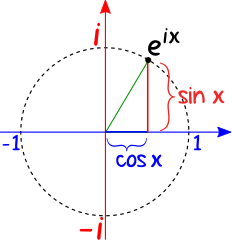# Euler's relation (e^(j*pi/4)

I am doing EE hw right now while going over my notes, I notice that my prof said that e^(jpi/4) = 1 but how? Using Euler's, I would get e(jpi/4) = cos(pi/4) + jsin(pi/4) = 0.7071 + j0.7071• Take the magnitude of cos(pi/4) + jsin(pi/4) and you'll get one. x+iy is a vector, it has direction and magnitude. 1 is just the magnitude only, 0.7+0.7j has a magnitude of one and an angle of 45'. Magnitude is sqrt(x^2 + y^2). – Sam May 5 '16 at 9:45
• Those vertical lInes mean magnitude – Scott Seidman May 5 '16 at 12:08
• The edits you have made to your question are wrong for two reasons. 1) you have tried to correct your numbers after I pointed out the problem and 2) the picture you have added (since my answer) is irrelevant. If you are going to edit a question following what is revealed in an answer, make sure you do it in a way that people reading my answer will not be confused into thinking I've missed the point. Common courtesy really. – Andy aka May 5 '16 at 13:57Hence $\sqrt{0.7071^2 + 0.7071^2}$ = 1
$$z = \alpha + j\beta = r e^{j\omega}$$ $$|z| = \sqrt{\alpha^2+\beta^2} =r$$ $$\angle z = \arg(z)=\omega=\arctan(\frac{\beta}{\alpha})$$